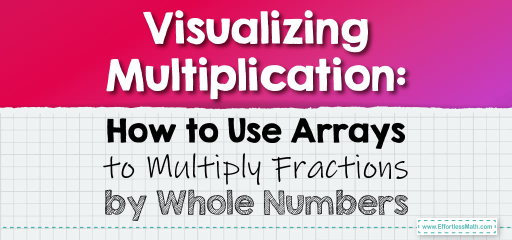# Visualizing Multiplication: How to Use Arrays to Multiply Fractions by Whole Numbers

Multiplying fractions by whole numbers can be visualized effectively using arrays.Arrays provide a clear and tangible representation of the multiplication process, making it easier to grasp, especially for visual learners. In this guide, we’ll delve into the method of using arrays to multiply fractions by whole numbers.

## Step-by-step Guide:

1. Understanding Arrays:

An array is a systematic arrangement of objects, usually in rows and columns. When dealing with fractions and whole numbers, arrays can be used to represent the whole number as rows and the fraction as a part of each row.

2. Setting Up the Array:

To multiply a fraction by a whole number:

– Draw rows corresponding to the whole number.

– Divide each row into equal parts based on the denominator of the fraction.

– Shade the number of parts indicated by the numerator of the fraction in each row.

Once the array is set up:

– Count the total number of shaded parts. This represents the product’s numerator.

– The denominator remains the same as the original fraction’s denominator.

4. Simplifying the Result:

If the result is an improper fraction, convert it to a mixed number for simplicity.

### Example 1:

Multiply $$\frac{2}{3}$$ by 4 using an array.

Solution:

– Draw 4 rows (for the whole number 4).

– Divide each row into 3 equal parts (for the denominator 3).

– Shade 2 parts in each row (for the numerator 2).

In total, 8 parts are shaded.

The result is $$\frac{8}{3}$$, which is equal to $$2 \frac{2}{3}$$.

The Absolute Best Book for 5th Grade Students

### Example 2:

Multiply $$\frac{3}{5}$$ by 3 using an array.

Solution:

– Draw 3 rows (for the whole number 3).

– Divide each row into 5 equal parts (for the denominator 5).

– Shade 3 parts in each row (for the numerator 3).

In total, 9 parts are shaded.

The result is $$\frac{9}{5}$$, which is equal to $$1 \frac{4}{5}$$.

### Practice Questions:

1. Multiply $$\frac{2}{4}$$ by 3 using an array.

2. Multiply $$\frac{3}{7}$$ by 5 using an array.

3. Multiply $$\frac{4}{6}$$ by 2 using an array.

A Perfect Book for Grade 5 Math Word Problems!

1. $$1 \frac{1}{2}$$ or $$1.5$$

2. $$2 \frac{1}{7}$$

3. $$1 \frac{1}{3}$$ or $$1.33…$$

The Best Math Books for Elementary Students

### What people say about "Visualizing Multiplication: How to Use Arrays to Multiply Fractions by Whole Numbers - Effortless Math: We Help Students Learn to LOVE Mathematics"?

No one replied yet.

X
51% OFF

Limited time only!

Save Over 51%

SAVE $15 It was$29.99 now it is \$14.99